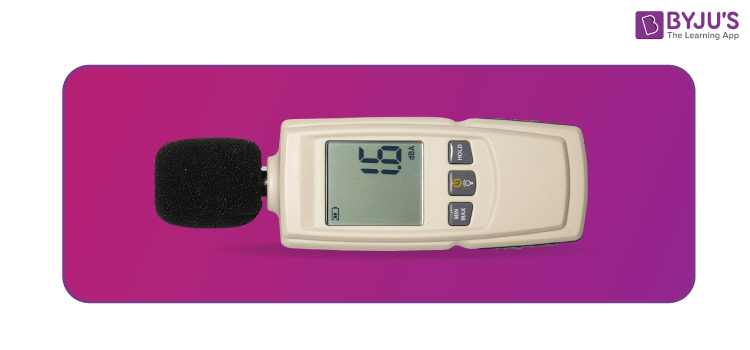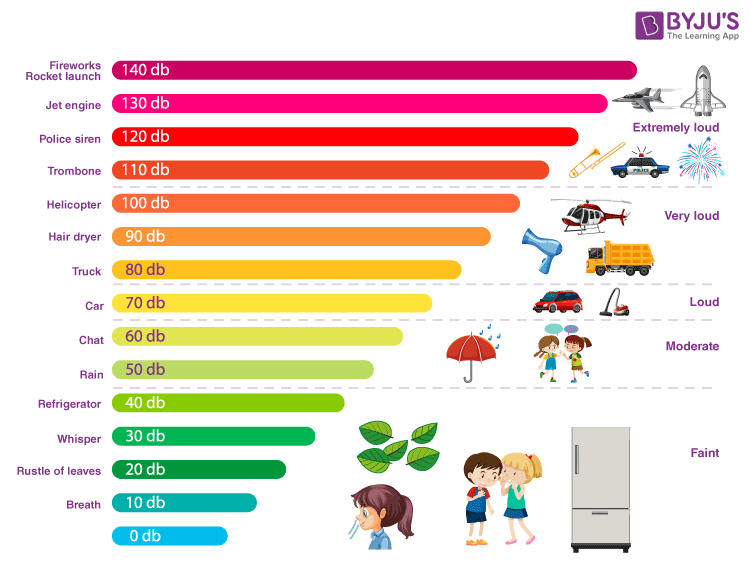# Decibel

The sound is all around us and can be measured to protect and inform us, as some sounds are not safe. In fact, loud noise can actually damage our hearing. So, the intensity of a sound is measured using decibel. As the human ear is incredibly sensitive, you can hear everything from your fingertip brushing lightly over your skin to a loud thunderclap. As far as power is concerned, the sound of the thunderclap is approximately 1,000,000,000,000 times more powerful than the smallest audible sound. That makes a huge difference! In this article, let us learn more about the unit decibel.

## What is Decibel?

Decibel is the unit of sound. Its symbol is ‘dB’. We can define decibel as

A unit of measurement used to express the ratio of one value of a power or field quantity to another on a logarithmic scale, the logarithmic quantity being called the power level or field level, respectively.

## Formulas to Calculate Decibel

The calculation of decibel can be done when power is given as well as when current and voltage are given.

• When power is given:

The most basic form for decibel calculations is given below:

 $$\begin{array}{l}N_{dB}=10log_{10}(\frac{P_{2}}{P_{1}})\end{array}$$
• When the voltage and current are given:
 $$\begin{array}{l}N_{dB}=20log_{10}(\frac{V_{2}}{V_{1}})\end{array}$$ $$\begin{array}{l}N_{dB}=20log_{10}(\frac{I_{2}}{I_{1}})\end{array}$$

You may also want to check out these topics given below!

## What is a Decibel Meter?

Sound can be measured with a device called a decibel meter. It measures and samples sound. Decibel meters are also known as sound-level meters. It can even be accessed on a smartphone through apps. Measuring the sound of an environment with a common device like a smartphone that many people always carry may help protect their ears more often.

• The decibel (dB) is a unit that expresses the ratio of two values of a physical quantity, often power or intensity.
• One decibel is one-tenth of one bel, a unit named in honour of Alexander Graham Bell. This unit ‘bel‘ is seldom used.### Decibel Meter Uses

A decibel meter is used for acoustic measurements. Sound travelling in acoustic means that the sound is travelling through the air. This instrument is usually a small device with a microphone that measures the noise. In acoustic, a diaphragm is a transducer intended to interconvert mechanical vibrations to sounds or vice versa. The diaphragm present in the microphone reacts to the changes in air pressure due to sound waves.

• This is another reason why this instrument is also referred to as a Sound Pressure Level (SPL meter). In the diaphragm movement, the sound pressure deviation is converted into an electrical signal (V).
• When constant pressure is applied, a microphone can be traced by the voltage produced, called the microphone sensitivity. The decibel meter uses the sensitivity of the microphone and converts the electrical signal accurately to sound pressure, and shows the resulting level of sound pressure (decibels dB).
• This instrument is usually used to measure noise and serves as a level meter. If human beings are exposed to loud noises for a brief period, it doesn’t cause much harm, but continuous exposure can do physical damage like eardrums damage.
• Sound level meters is mostly used in noise pollution studies to analyze the quantification of various kinds of noise. Thus, this device helps to measure the intensity of the sound, which can measure the decibel of the sound so that one can take effective measures to reduce it.

## What is the Decibel Scale?

The human ear has the ability to handle an immense range of different sound levels. Therefore, a logarithmic scale is used to express sound levels meaningfully in more flexible numbers rather than a linear one. This scale is called the decibel scale or dB scale.

• On the decibel scale, 0 dB is the smallest audible sound.
• A sound 10 times more powerful is 10 dB.
• A sound 100 times more powerful than near total silence is 20 dB.
• A sound 1,000 times more powerful than near total silence is 30 dB.Decibel Scale or DB Scale.

### Noise Level Chart (Decibel Chart)

A noise level chart showing examples of sounds with dB levels ranging from 0 to 120 decibels is given below.

 Decibel Sound Source 10 Almost inaudible Normal breathing 20 Audible Rustling leaves, Mosquitoes 30 Very quiet Whisper 40 Quiet Stream, Refrigerator humming 50 Limited Sound Quiet office 55 Normal Sound Filtering Coffee maker 60 Fairly Quiet Normal conversation 70 Irritating Vacuum cleaner, Hairdryer 75 Constant Sound Dishwasher 80 Unpleasant City traffic noise 85 Loud Lawnmower 90 Louder Violin 95 Noisy Farm tractor 100 Extremely Unpleasant Train 105 Even Louder Large drum 110 Extremely Loud Symphony orchestra 120 Extremely Loud Thunderclap, Boombox### Why do we use Decibel (dB)?

1. The decibel is mostly applied in acoustics.
2. It is used as a unit of sound pressure level. In this, the reference pressure in air is set as the threshold value of the perception of a human. Many comparisons are used to describe separate levels of pressure.
3. The decibel is used to express the ratios of power or amplitude in arithmetic ratios in electronics. The total gain of a decibel can be easily calculated by adding up all the decibel gains.